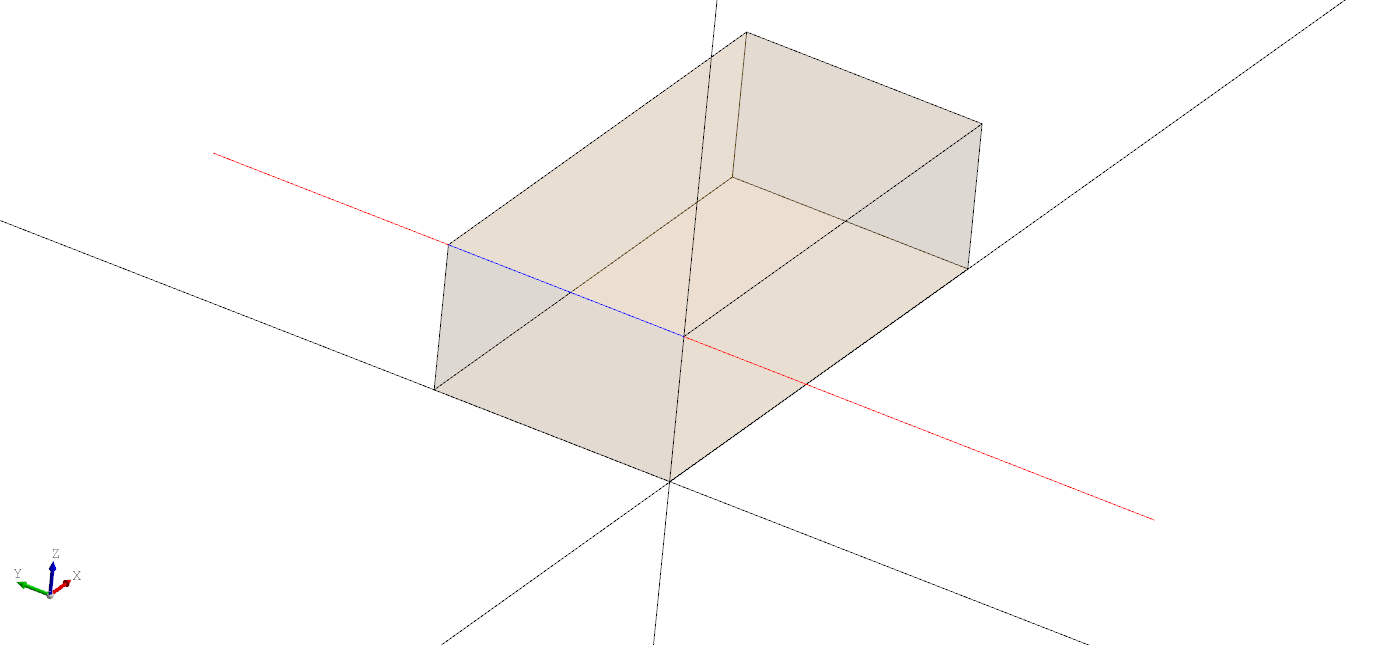The `TrimmedCurve` declaration can be used to reparametrize an edge of a shape to shorten or extend it. To use it either set the `shape` to the edge you want to reparametrize or embed another curve within the node. Then set the new `u` and `v` values for the start and end parameters.

Note: The current parameters of a curve can be obtained by using `Topology(shape=edge).curve_bounds` which returns the min and max of the curve.

``````# Created in DeclaraCAD

enamldef Assembly(Part):
Axis:
pass
Box: segment:
transparency = 0.8
dx = 10
dy = 5
dz = 3
TrimmedCurve:
color = 'red'
u = -10
v = 10
shape = segment.topology.edges
TopoShape:
color = 'blue'
shape = segment.topology.edges
``````